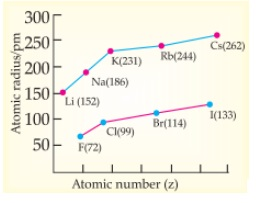Case Based Questions (MCQ)

Class 10
Chapter 3 Class 10 - Metals and Non-Metals

## Non-Metallic Character:  The ability of an atom to accept electrons to form  a negative ion (anion) is called non-metallic  character or electronegativity. The elements having  high electro-negativity have a higher tendency to gain electrons and form anion.  Down the group, electronegativity decreases due  to increase in atomic size and across the period,  from left to right electro -negativity increases due  to decrease in atomic size.### (iv) Cs>K>Rb>Na>Li

As we go down the group , atomic size increases and the ability to donate electrons, i.e. electropositivity increases . So, the metallic character also increases.

The order of increasing atomic size is as follows: Cs>Rb>K>Na>Li

So, the correct option is (iii)

### (iv) as Hydrogen shows the properties of nonmetals.

Alkali metals lose one electron to form a positive ion.

Hydrogen also loses its one electron to form H +

So, the correct answer is (ii)

### (iv) I

• Electronegativity decreases  as we go down the group.
• The order of electronegativity can be given as: F>Cl>Br>I

So, the correct answer is (i)

### (iv) Electronegativity increases down the group  due to increase in forces of attractions between  nucleus & valence electrons.

• Electronegativity decreases down the group due to an increase in atomic radius.
• It leads to a decrease in the force of attraction between valence electrons and nucleus.
• So, the tendency to gain electrons decreases

So, the correct answer is (III)

### (iv) F and Li are in the same period and across the  period atomic size/radius decreases from left  to right.

• F and Li are in the same period
• Atomic size/radius decreases from left to  right.

So, the correct answer is (III)

Get live Maths 1-on-1 Classs - Class 6 to 12